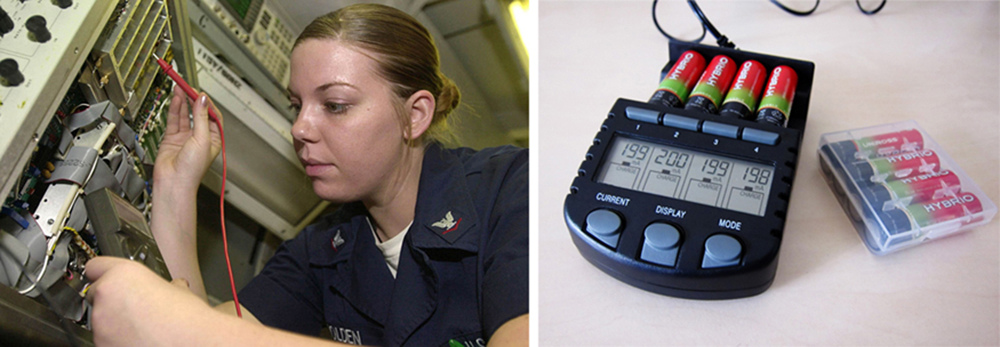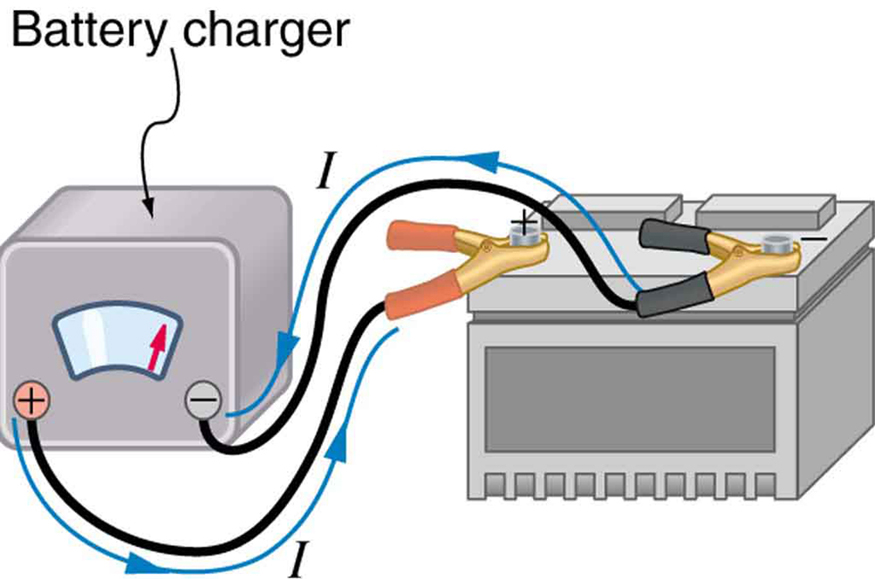# 21.2 Electromotive force: terminal voltage  (Page 4/12)

 Page 4 / 12
$\begin{array}{lll}V& =& \text{emf}-\text{Ir}=\text{12.0 V}-\left(\text{1.188 A}\right)\left(\text{0.100 Ω}\right)\\ & =& \text{11.9 V.}\end{array}$

Discussion for (a)

The terminal voltage here is only slightly lower than the emf, implying that $\text{10}\text{.}0\phantom{\rule{0.25em}{0ex}}\Omega$ is a light load for this particular battery.

Solution for (b)

Similarly, with ${R}_{\text{load}}=0\text{.}\text{500}\phantom{\rule{0.15em}{0ex}}\Omega$ , the current is

$I=\frac{\text{emf}}{{R}_{\text{load}}+r}=\frac{\text{12}\text{.}0\phantom{\rule{0.25em}{0ex}}\text{V}}{0\text{.}\text{600}\phantom{\rule{0.15em}{0ex}}\Omega }=\text{20}\text{.}0\phantom{\rule{0.25em}{0ex}}\text{A}.$

The terminal voltage is now

$\begin{array}{lll}V& =& \text{emf}-\text{Ir}=\text{12.0 V}-\left(\text{20.0 A}\right)\left(\text{0.100 Ω}\right)\\ & =& \text{10}\text{.}\text{0 V.}\end{array}$

Discussion for (b)

This terminal voltage exhibits a more significant reduction compared with emf, implying $0\text{.}\text{500}\phantom{\rule{0.15em}{0ex}}\Omega$ is a heavy load for this battery.

Solution for (c)

The power dissipated by the $0\text{.}\text{500 - Ω}$ load can be found using the formula $P={I}^{2}R$ . Entering the known values gives

${P}_{\text{load}}={I}^{2}{R}_{\text{load}}={\left(\text{20.0}\phantom{\rule{0.25em}{0ex}}\text{A}\right)}^{2}\left(0\text{.500 Ω}\right)=2.00×{10}^{2}\phantom{\rule{0.25em}{0ex}}\text{W}.$

Discussion for (c)

Note that this power can also be obtained using the expressions $\frac{{V}^{2}}{R}$ or $\text{IV}$ , where $V$ is the terminal voltage (10.0 V in this case).

Solution for (d)

Here the internal resistance has increased, perhaps due to the depletion of the battery, to the point where it is as great as the load resistance. As before, we first find the current by entering the known values into the expression, yielding

$I=\frac{\text{emf}}{{R}_{\text{load}}+r}=\frac{\text{12}\text{.}0\phantom{\rule{0.25em}{0ex}}\text{V}}{1\text{.}\text{00}\phantom{\rule{0.25em}{0ex}}\Omega }=\text{12}\text{.}0\phantom{\rule{0.25em}{0ex}}\text{A}.$

Now the terminal voltage is

$\begin{array}{lll}V& =& \text{emf}-\text{Ir}=\text{12.0 V}-\left(\text{12.0 A}\right)\left(\text{0.500 Ω}\right)\\ & =& \text{6.00 V,}\end{array}$

and the power dissipated by the load is

${P}_{\text{load}}={I}^{2}{R}_{\text{load}}={\left(\text{12.0 A}\right)}^{2}\left(0\text{.}\text{500}\phantom{\rule{0.25em}{0ex}}\Omega \right)=\text{72}\text{.}0\phantom{\rule{0.25em}{0ex}}\text{W}.$

Discussion for (d)

We see that the increased internal resistance has significantly decreased terminal voltage, current, and power delivered to a load.

Battery testers, such as those in [link] , use small load resistors to intentionally draw current to determine whether the terminal voltage drops below an acceptable level. They really test the internal resistance of the battery. If internal resistance is high, the battery is weak, as evidenced by its low terminal voltage.These two battery testers measure terminal voltage under a load to determine the condition of a battery. The large device is being used by a U.S. Navy electronics technician to test large batteries aboard the aircraft carrier USS Nimitz and has a small resistance that can dissipate large amounts of power. (credit: U.S. Navy photo by Photographer’s Mate Airman Jason A. Johnston) The small device is used on small batteries and has a digital display to indicate the acceptability of their terminal voltage. (credit: Keith Williamson)

Some batteries can be recharged by passing a current through them in the direction opposite to the current they supply to a resistance. This is done routinely in cars and batteries for small electrical appliances and electronic devices, and is represented pictorially in [link] . The voltage output of the battery charger must be greater than the emf of the battery to reverse current through it. This will cause the terminal voltage of the battery to be greater than the emf, since $V=\text{emf}-\text{Ir}$ , and $I$ is now negative.A car battery charger reverses the normal direction of current through a battery, reversing its chemical reaction and replenishing its chemical potential.

## Multiple voltage sources

There are two voltage sources when a battery charger is used. Voltage sources connected in series are relatively simple. When voltage sources are in series, their internal resistances add and their emfs add algebraically. (See [link] .) Series connections of voltage sources are common—for example, in flashlights, toys, and other appliances. Usually, the cells are in series in order to produce a larger total emf.

derivative of first differential equation
why static friction is greater than Kinetic friction
draw magnetic field pattern for two wire carrying current in the same direction
An American traveler in New Zealand carries a transformer to convert New Zealand’s standard 240 V to 120 V so that she can use some small appliances on her trip.
What is the ratio of turns in the primary and secondary coils of her transformer?
nkombo
How electric lines and equipotential surface are mutually perpendicular?
The potential difference between any two points on the surface is zero that implies È.Ŕ=0, Where R is the distance between two different points &E= Electric field intensity. From which we have cos þ =0, where þ is the angle between the directions of field and distance line, as E andR are zero. Thus
sorry..E and R are non zero...
By how much leeway (both percentage and mass) would you have in the selection of the mass of the object in the previous problem if you did not wish the new period to be greater than 2.01 s or less than 1.99 s?
what Is linear momentum
why no diagrams
where
Fayyaz
Myanmar
Pyae
hi
Iroko
hello
Abdu
Describe an experiment to determine short half life
what is science
it's a natural phenomena
Hassan
sap
Emmanuel
please can someone help me with explanations of wave
Benedine
there are seven basic type of wave radio waves, gyamma rays (nuclear energy), microwave,etc you can also search 🔍 on Google :-)
Shravasti
A 20MH coil has a resistance of 50 ohms and us connected in series with a capacitor to a 520MV supply
what is physics
it is the science which we used in our daily life
Sujitha
Physics is the branch of science that deals with the study of matter and the interactions it undergoes with energy
Junior
it is branch of science which deals with study of happening in the human life
AMIT
A 20MH coil has a resistance of 50 ohms and is connected in series with a capacitor to a 250MV supply if the circuit is to resonate at 100KHZ, Determine 1: the capacitance of the capacitor 2: the working voltage of the circuit, given that pie =3.142
Musa
Physics is the branch of science that deals with the study of matter and the interactions it undergoes with energy
Kelly
Heat is transfered by thermal contact but if it is transfered by conduction or radiation, is it possible to reach in thermal equilibrium?
Yes, It is possible by conduction if Surface is Adiabatic
Astronomy
Yeah true ilwith d help of Adiabatic
Kelly
what are the fundamentals qualities
what is physic3
Kalilu
what is physic
Kalilu
Physics? Is a branch of science dealing with matter in relation to energy.
Moses
Physic... Is a purging medicine, which stimulates evacuation of the bowels.
Moses
are you asking for qualities or quantities?
Noman
fundamental quantities are, length , mass, time, current, luminous intensity, amount of substance, thermodynamic temperature.
Shravasti
fundamental quantities are quantities that are independent of others and cannot be define in terms of other quantities there is nothing like Qualities we have only fundamental quantities which includes; length,mass,time, electric current, luminous density, temperature, amount of substance etc
give examples of three dimensional frame of reference
Universe
Noman
Yes the Universe itself
AstronomyByByBy Savannah ParrishBy Katie MontroseByBy JavaChamp TeamBy OpenStaxBy OpenStaxBy OpenStaxBy Anonymous UserBy OpenStaxBy Yasser Ibrahim